## Example Questions

1 2 4 Next →

### Example Question #671 : Sat Mathematics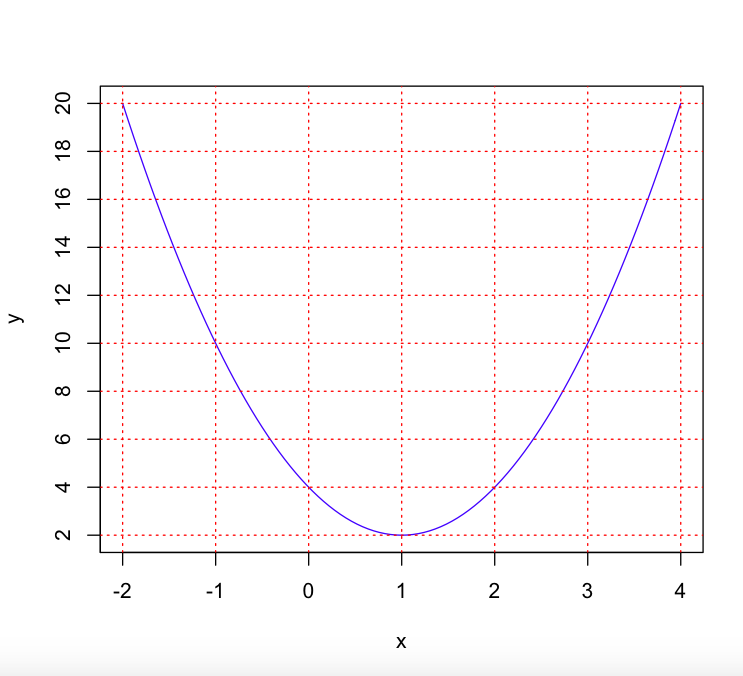What is the equation of the graph?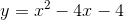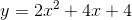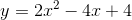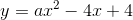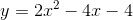Explanation:

In order to figure out what the equation of the image is, we need to find the vertex. From the graph we can determine that the vertex is at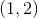. We can use vertex form to solve for the equation of this graph.

Recall vertex form,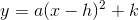, whereis thecoordinate of the vertex, andis thecoordinate of the vertex.

Plugging in our values, we get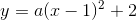To solve for, we need to pick a point on the graph and plug it into our equation.

I will pick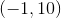.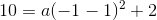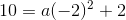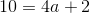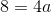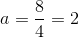Now our equation is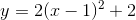Let's expand this,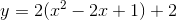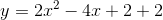### Example Question #672 : Sat Mathematics

How many times does the equation below cross the x-axis?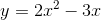Explanation:

You can solve this problem two ways.

3. You can solve for where the graph crosses the x-axis by setting the equation equal to zero, factoring, and solving.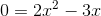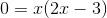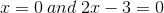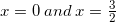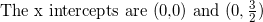2. You can quickly sketch the graph by choosing some x values and solving for y.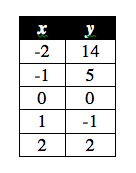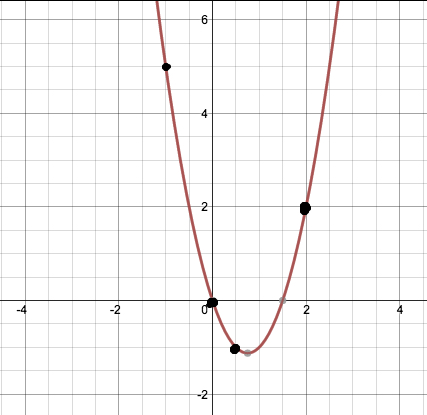We see that the graph passes the x-axis twice.

### Example Question #676 : Sat Mathematics

Let f(x) = x2. By how many units must f(x) be shifted downward so that the distance between its x-intercepts becomes 8?

12

8

4

16

2

16

Explanation:

Because the graph of f(x) = x2 is symmetric about the y-axis, when we shift it downward, the points where it intersects the x-axis will be the same distance from the origin. In other words, we could say that one intercept will be (-a,0) and the other would be (a,0). The distance between these two points has to be 8, so that means that 2a = 8, and a = 4. This means that when f(x) is shifted downward, its new roots will be at (-4,0) and (4,0).

Let g(x) be the graph after f(x) has been shifted downward. We know that g(x) must have the roots (-4,0) and (4,0). We could thus write the equation of g(x) as (x-(-4))(x-4) = (x+4)(x-4) = x2 - 16.

We can now compare f(x) and g(x), and we see that g(x) could be obtained if f(x) were shifted down by 16 units; therefore, the answer is 16.

1 2 4 Next →

### All SAT Math Resources